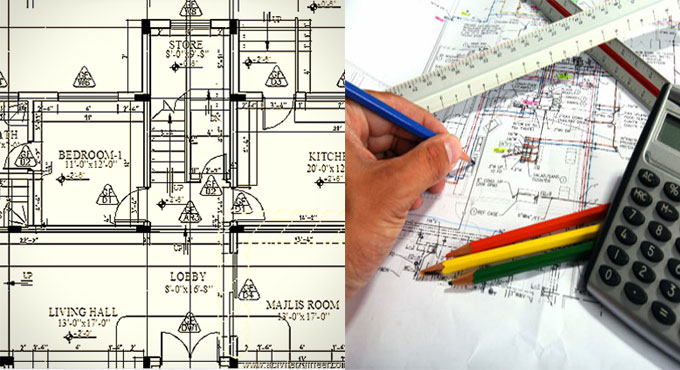# Types of Estimate found on various stages of a ProjectEstimate is a raw calculation on quantities of various works and their expenses which are made by the experts of the proper field before a project gets complete. Accuracy of an estimate depends on the experience, ability and judgment power of the estimator.

An estimator has to be very careful about the quantity takeoff using the given data such as drawings, specifications and other documents about the project. Besides all of this, every estimate must be prepared with the consideration of other two factors which influence a project namely Direct & Indirect Cost. Direct Cost includes cost of materials, equipment, labor and subcontracted cost; while the Indirect cost includes overheads and contingency.

Types of estimate: There are seven types of estimates which are described below:

1. Approximate estimate: It is also known as budget, preliminary estimate which is prepared in the first stage of a project; this estimation is made to give a clear idea to the client about the amount of cost needed for the project and to get the acceptance from necessary approving bodies or banks to get the loan. So mainly the approximate estimate is calculated with relevant previous experience and the documents like project drawing plans, details about land, electricity & water supply and a full detailed report are necessary to make the estimate. So, Approximate estimate = rate of 1m2

2. Plinth Area estimate: This can be calculated by multiplying the values of length, width and area of plinth. The plinth area is considered as additional plinth area of the building at floor level which can also be known as the roof covered area of a building. The plinth area rate is originated by dividing the total cost of an early constructed building by plinth area of the earlier constructed building.

3. Cubic content estimate: It is done by multiplying the volume of the building by the unit cubic rate got form the earlier estimate.

4. Annual repair estimate and special repair estimate: These kinds of estimates are calculated for managing the constructed element in good condition. Special repair estimate is made in situations where the costs of materials increased when compare to annual repair estimate cost.

5. Revised estimate: This is made when the rate of earlier submitted estimate rises by 5% or more than that. There can be many reasons for the preparation of estimate as per the increase cost of materials while the reason and comparative statement between two estimates can be joined with the revised estimate.

6. Supplementary estimate: It is made when there is an important situation of supplementary work to progress out the original work.

7. Detailed estimate: This type of estimate is made with the help of complete set of contract data and the making of detailed estimate can do under two phrases like work out with quantities of different works and calculate the cost of each work. A detailed estimate must have some documents like report, specifications, drawings/plans, design charts and schedule of rates. Besides these there are many other factors that are needed to consider well in the time of planning the detailed estimate.

Article Source: www.basiccivilengineering.com